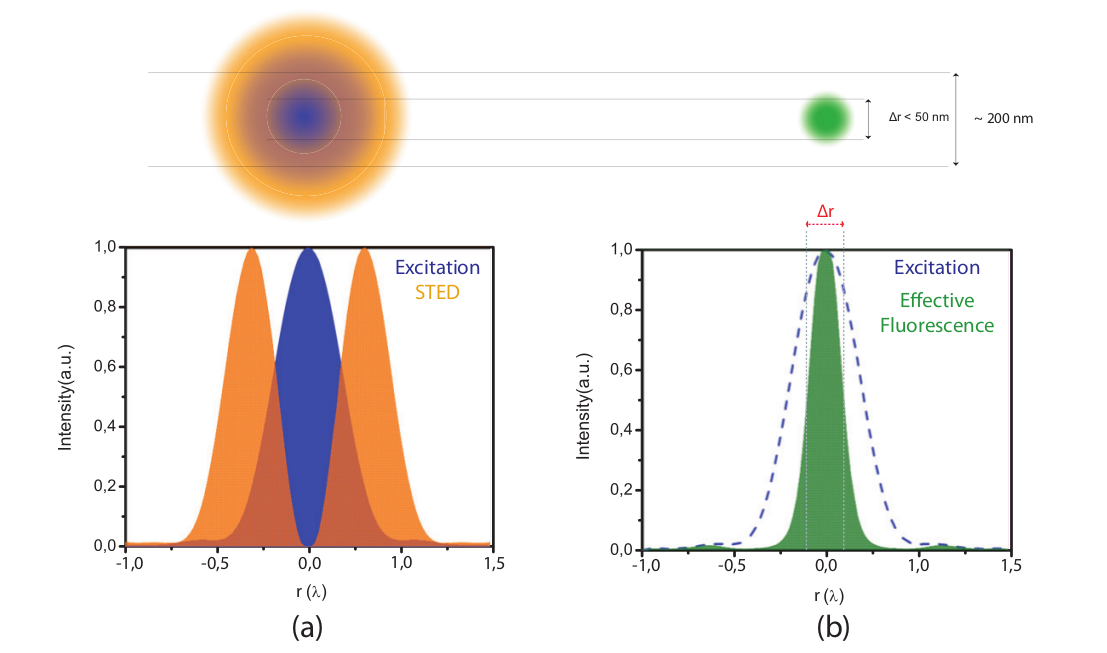# STED Resolution: mathematical derivation of the STED equation

The increase in spatial resolution in STED microscopy is dependent on the STED depletion intensity and can be described mathematically. This page gives the mathematical derivation of the inverse-square-root STED formula:(10)

Letbe the normalized excitation probability in the focal plane resulting from the excitation pulse. After the passage of a STED pulse, the fluorescence probabilityof a molecule has decayed to(1)

with,anddenoting the cross section for stimulated emission, STED pulse duration and the focal intensity of the STED pulse, respectively. Note thather is given by number of photons per unit area per unit time.
As a result of the overlapping STED beam, the probability to detect a photon from locationis proportional to(2)

Given a lens with semi aperture, we can describe the spatial excitation probabilityand the STED intensityby:(3)(4)

Normalized cross sections ofandare also illustrated in figure 1 (a) as the blue curve and yellow curve respectively.is simply the maximum STED intensity in the doughnut shape. For further simplification we can define the constantas(5)

If we combine equations (1), (3), (4) and (5) with equation (2), and use the approximation, equation (2) can be written as:(6)is the FWHM of the effective fluorescent spot, which cross section is also represented as the green curve in Figure 1 (b). We can further approximate equation (6) with a Taylor seriesto the second order:(7)

At the FWHM we have, so we can solve for:(8)

The FWHMcan then be described with:(9)

From equation (1) we can see that forthe fluorescence has dropped toof the initial value. By introducingin equation (9) we obtain:(10)

Equation (10) is the familiar inverse square root intensity law for STED: the FWHM resolution approximately scales with the inverse square root of the STED intensity. In case of gated-STED there is an additional factor that takes the effect of gating into account, leading to an additional resolution increase.Figure 1. Top: overlapping a doughnut-shaped STED spot with a diffraction limited excitation spot will result in a confinement of the area in which fluorophores are able to fluoresce. Bottom: (a) Graph illustrating the overlap of the intensity of the STED donut shaped spot I_STED (r) (orange) and excitation spot (blue) in one dimension. (b) Graph illustrating the decrease in the width of the effective fluorescence probability h(r) (green) as a result of STED. Δr is the FWHM of the effective fluorescence probability (equation 8.3.)

 Remko R.M. Dijkstra, Design and realization of a CW-STED super-resolution microscope setup, Master Thesis , University of Twente, 2012
 Volker Westphal and Stefan W. Hell, ''Nanoscale Resolution in the Focal Plane of an Optical Microscope, Phys. Rev. Lett. 94, 143903, April 2005